# Quiz 13: Risk Analysis

The IRR (Internal Rate of return) can be defined as the rate of return at which the present value of outflows on the investment will be equal to the present value of inflows from the investment. The After-Tax IRR is computed on the after-tax cash flows from the investment, and the before-tax IRR (BTIRR) is computed on the before-tax cash flows. a. Use the spreadsheet "IRR" function to compute the BTIRR for this case. Enter values and formulas in the spreadsheet as shown in the image below.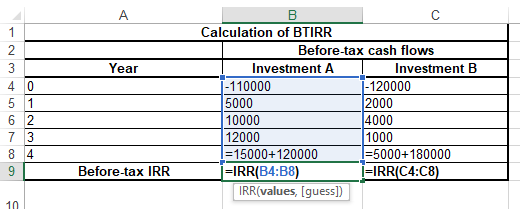Here, the cash flow in year-4 is the sum of operating cash flows and cash flow from sales. The obtained result is provided below.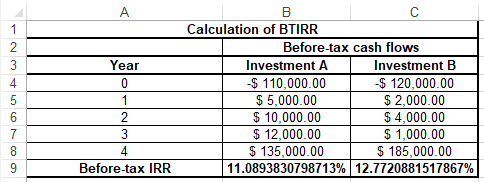Thus, the BTIRR for Investment A and Investment B are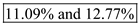respectively. b. The requirement is to partition the before-tax cash flows in to the operating cash flows and the cash flow from sales to know the contribution of each proportion in BTIRR. Use the spreadsheet to know the contribution of each in BTIRR. Enter values and formulas in the spreadsheet as shown in the image below.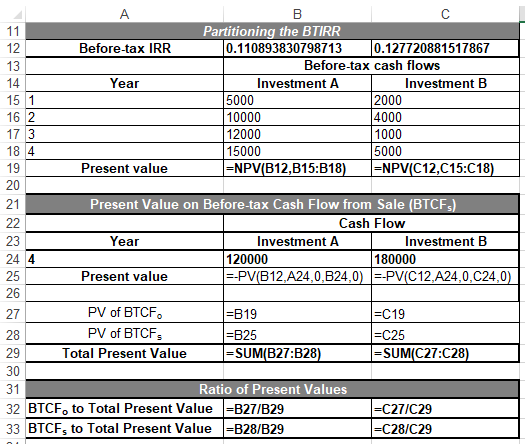Obtained result is provided below.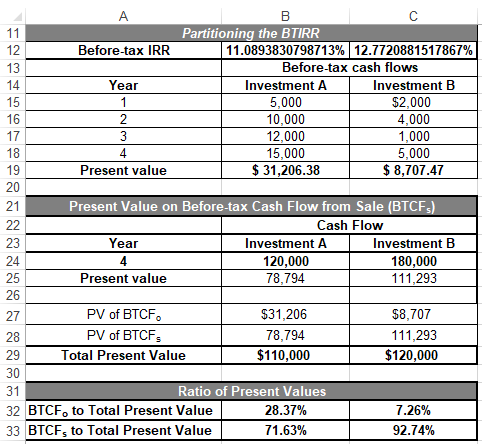Thus, the contribution of before-tax cash flow from operation and cash flow from sale in BTIRR for Investment A are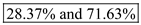respectively. Thus, the contribution of before-tax cash flow from operation and cash flow from sale in BTIRR for Investment B are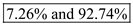respectively. c. The proportion of contribution made by operating cash flows and the cash flow from sales indicate that how stable the cash flows are. It is assumed that the more contribution of operating cash flow in BTIRR indicate that cash flows are more certain. For the given case the contribution of operating cash flows in BTIRR of Investment A is the highest. Thus, it could be concluded that the cash flows of Investment A are more certain compared to cash flows of Investment B. It is also evident from the calculation part b that in BTIRR the major contribution is of cash flow from sales for both investments. Thus, the BTIRR of both investments is vulnerable.
Internal rate of return (IRR): It is the rate at which the sum of cash outflows and cash inflow values becomes zero at initial year. This rate helps to determine the selection of a project. If the internal rate of return of a project comes higher than the company's required rate of return, then company takes the project. Computation of expected return: Expected return can be computed with the help of estimated before tax internal rate of return (BTIRR) and the probability percentage in each state of economy. For Investment I: Estimated BTIRR for investment I in optimistic state of economy is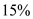, in most likely state is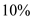, and in pessimistic state is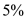. Probability percentage in optimistic state is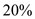, in most likely state is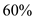, in pessimistic state isCalculation of expected return is given in below excel.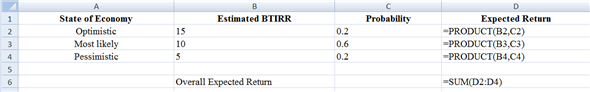Fig (1) The resultant figure from the excel sheet is given below.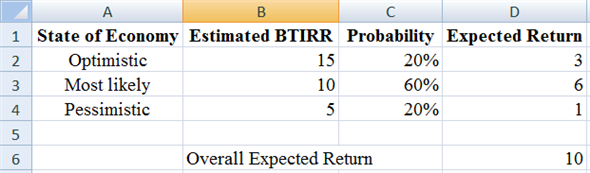Fig (2) Computation of expected return: Expected return can be computed with the help of estimated before tax internal rate of return (BTIRR) and the probability percentage in each state of economy. For Investment II: Estimated BTIRR for investment II in optimistic state of economy is, in most likely state is, and in pessimistic state is. Probability percentage in optimistic state is, in most likely state is, in pessimistic state isCalculation of expected return is given in below excel.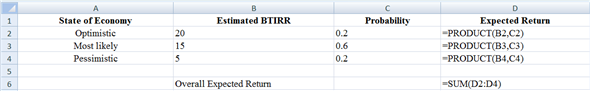Fig (3) The resultant figure from the excel sheet is given below.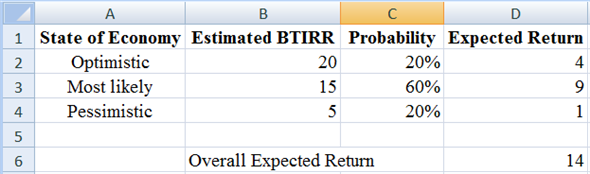Fig (4) Hence, the expected return for investment I isand of investment II is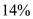Computation of variance and standard deviation: Calculate the difference between estimated and expected return, square the resultant deviation, then multiply it with the probability percentage and at last sum of all the product will be the variance. To calculate the standard deviation, under root the value of variance. For Investment I: Estimated BTIRR for investment I in optimistic state of economy is, in most likely state is, and in pessimistic state is. Probability percentage in optimistic state is, in most likely state is, in pessimistic state isCalculation of variance and standard deviation is given in below excel.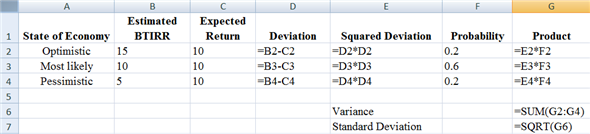Fig (5) The resultant figure from the excel sheet is given below.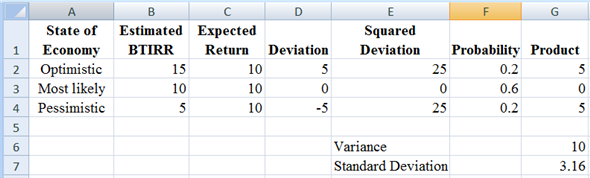Fig (6) For Investment II: Estimated BTIRR for investment II in optimistic state of economy is, in most likely state is, and in pessimistic state is. Probability percentage in optimistic state is, in most likely state is, in pessimistic state isCalculation of variance and standard deviation is given in below excel.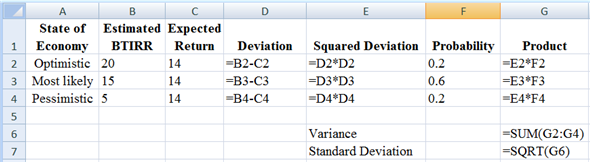Fig (7) The resultant figure from the excel sheet is given below.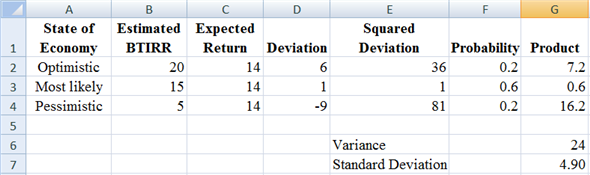Fig (8) Hence, the variance and standard deviation for investment I is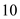and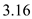respectively, and of investment II is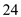and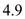respectively. Comparison between Investment I and II: The projected BTIRR is higher for Investment II by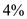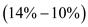. Standard deviation is moreover high for investment II vs. investment I by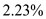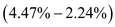. According to the calculated data, Investment II has a higher expected BTIRR but is also riskier. It cannot be concluded that which among these two investments is better one. It can be said that Investment II dominates Investment I because in none of the scenario the returns of Investment II are lower than Investment I.